# Harmonicaly Excited Forced Vibration of a Single DOF System

2.3 Differential equation for Forced vibration

A single DOF system with viscous damping, excited by a harmonic force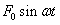is shown in Fig. 2.1.

###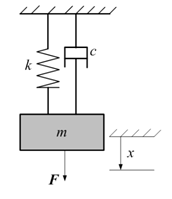Fig. 2.1: Viscously damped system with harmonic excitation

Its differential equation of motion is: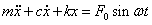(2.1)

The general solution of this mathematical model consists of two parts, the complementary function which is the solution of the homogenous equation, and the particular integral.

The particular solution to the preceding equation is a steady-state oscillation of same frequency ω  as that of excitation. We can assume particular solution to be of the form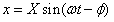(2.2)

Where X is the amplitude of oscillation and  Φ is the phase of the displacement with respect to the exciting force.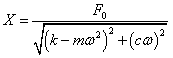(2.3)

And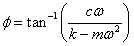(2.4)

Now, dividing the numerator and denominator of equation (2.3)  and (2.4) by k , we obtain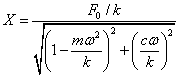(2.5)

And,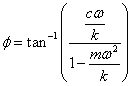(2.6)

The above equations may be represented in terms of following quantities:

ω nf = √(k/m) = natural frequency of un-damped oscillation

c c = 2mω nf  = critical damping constant

ζ = c/c c = critical damping ratio

###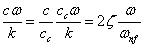The non-dimensional expression for the amplitude and phase then become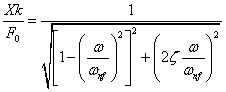(2.7)

And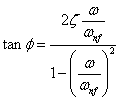(2.8)

These equations indicate that the non-dimensional amplitude Xk/F 0 , termed as magnification factor and the phase Ø are functions of the frequency ratio ω/ω nf  and the damping factor ζ and can be plotted as shown in Fig. 2.2 & Fig. 2.3. These curve show that the damping factor has a large influence on the amplitude and phase angle in the frequency region near resonance. The curves show that as the damping ratio increases, the maximum value of magnification factor decreases. When there is no damping i.e. (ζ=0), it reaches to infinity at the resonance i.e. when ω/ω nf = 1.

###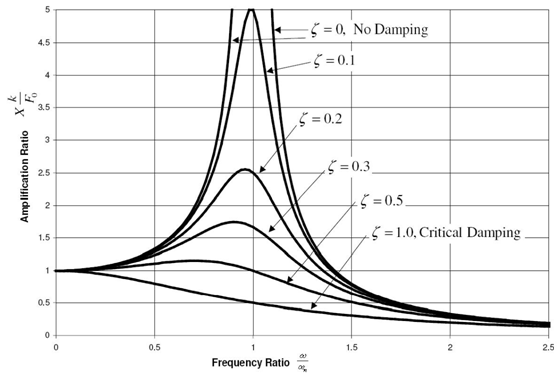Fig. 2.2:    Magnification factor versus frequency ratio for different values of damping ratio

###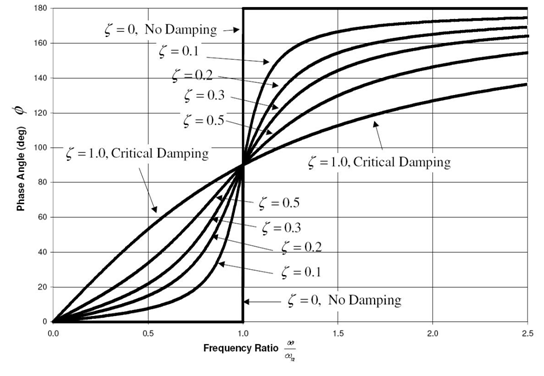Fig. 2.3: Phase angle versus frequency ratio for different values of damping ratio